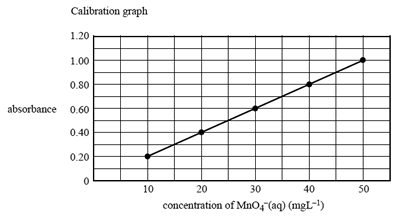Gravimetric analysis exercises (2008 VCE) One method of analysing the manganese content of steel is to dissolve the steel in nitric acid; producing a solution of manganese(II) ions, Mn2+(aq). The Mn2+((aq) ions are then treated with an excess of acidified solution of periodate ions, IO4-(aq). The products of this reaction are iodate ions, IO3- (aq), and the deeply purple-coloured permanganate ions, MnO4- (aq). The concentration of MnO4- (aq) is then determined by UV-visible spectroscopy. Calculate the oxidation number of iodine in the IO4-(aq) ion. Solution Give a half equation for the conversion of IO4-(aq) into IO3- (aq) in acid solution. Solution. Is the IO4-(aq) ion acting as an oxidant or reductant? Explain your choice. SolutionAn experiment was carried out to determine the percentage of manganese in a particular sample of steel by the above method. A 13.936 g sample of steel was dissolved in acid and the manganese was converted to MnO4-(aq) ions. The solution containing the MnO4-(aq) ions was fi ltered and made up to a volume of 1.00 L. 25.00 mL of this solution was then further diluted to 100.0 mL in a volumetric fl ask. The absorbance, at 525 nm, of this solution was 0.70. Next, the absorbance, at 525 nm, of a series of solutions of MnO4-(aq) ions of known concentration was measured and a calibration graph drawn.What is the concentration, in mg L–1, of MnO4-(aq) in the diluted solution in the 100 mL volumetric flask? Solution Calculate the mass, in mg, of manganese in the steel sample. Solution Calculate the percentage, by mass, of manganese in the steel sample. Solution